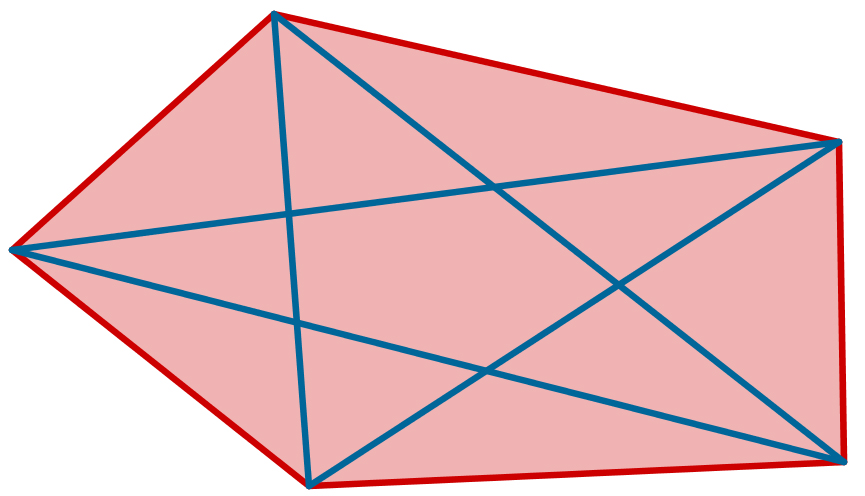# Pentagon

Geometry Level 3

Let $p$ denote the perimeter of a convex pentagon, and let $d$ denote the sum of diagonals of this same pentagon.

Is it true that for any convex pentagon, $p < d < 2p$ is fulfilled?×